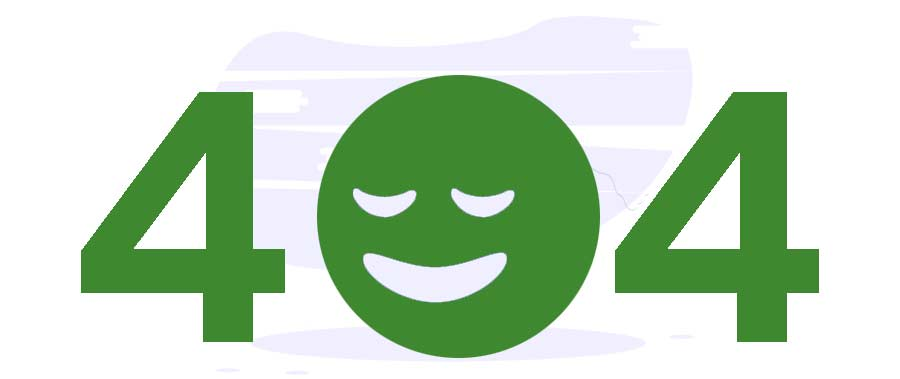# Narayana number in C++

Site Under Maintenance - Tutorialspoint# Error. We can’t find the page you’re looking for.

Sorry for the inconvenience. Go to our homepage or check out our Latest Courses, eBooks, Prime Packs...

Back to Home

The Narayana numbers can be expressed in terms of binomial expression $1/n\binom{n}{k} \binom{n}{k-1}$  Learn more about the Narayana number here.

You are given the numbers n and k. Find the Narayana number. It's a straightforward problem having the combinations formula. Let's see the code.

## Algorithm

• Initialise the numbers n and k.
• Find the Narayana number using the given formula.
• Print the resultant number.

## Implementation

Following is the implementation of the above algorithm in C++

#include <bits/stdc++.h>
using namespace std;
int factorial(int n) {
int product = 1;
for (int i = 2; i <= n; i++) {
product *= i;
}
return product;
}
int nCr(int n, int r) {
return factorial(n) / (factorial(n - r) * factorial(r));
}
int main() {
int n = 8, k = 5;
cout << nCr(n, k) * nCr(n, k - 1) / n << endl;
return 0;
}

## Output

If you run the above code, then you will get the following result.

490